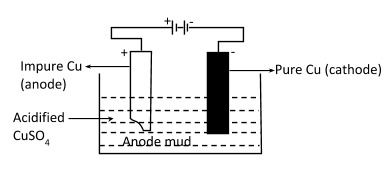Outline the principles of refining of metals by the following methods:(i) Zone refining(ii) Electrolytic refining(iii) Vapor phase refiningVerified
147.6k+ views
Hint: Zone refining: It is used for refining $Si,\,B,\,Ga,\,In,$ etc. impurities are more soluble in molten states of metal than in solid states.
>Electrorefining: It is carried out for refining of impure zinc. The anode is impure zinc and cathode is pure zinc.
>Vapor phase refining: Metal is converted into volatile compound and then decomposed to obtain pure metal.

(i) Zone refining: Semiconductors like $Si,Ge,Ga,$ etc. are purified by this method. Impurities are highly soluble in molten metal than in solid metal. This method is based on fractional crystallization. A circular heater surrounds a rod of impure metal. The heater is moved then the pure metal crystallizes out and impurities pass into the adjacent molten metal. The metal is repeated to shift the impurities to one end which is cut off.
$At{\text{ }}anode\,\mathop {Cu}\limits_{impure} \to \mathop {Cu}\limits^{ + 2} + \mathop {2e}\limits^ - \\ At{\text{ cathode}}\,\mathop {Cu}\limits^{ + 2} + \mathop {2e}\limits^ - \to \mathop {Cu}\limits_{pure} \\$